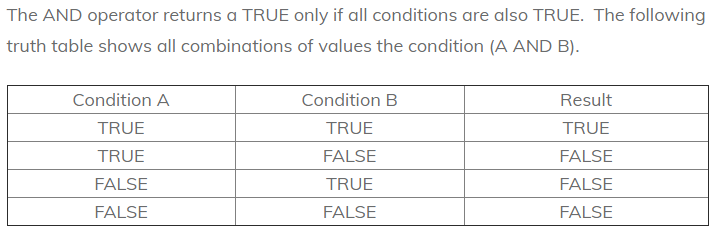Closed Loop Interval OntologyCLOSED LOOP INTERVAL ONTOLOGY        The Digital Integration of Conceptual FormTzimTzum/Kaballah | Loop definition | Home | ORIGINPlease sign in or register Email * Password * Searches selected display Universal conceptual form Aligning the vision Evolving and coalescing Why we do this Ethics / governance / science Homeostatic governance Idealized democracy Reconciliation and integration Holistic view on alternatives Definitions and alternatives Compare alternatives How do we know? How meaning is created Foundational recursive definition Spectrum of levels The closed loop ensemble contains all primary definitions Dimensions of set theory What is a number? Topology of sets How are they constructed? Primary terms Closed Loop framework Hierarchical models Euclid in digital space Foundational method Compositional semantics How it works The integrated science of mind What does it mean? Core terms Constructive elements and building blocks Preserving data under transformation In the beginning Domain and universal A design proposal Mapping the grid From other sources Foundational computation Perfecting political balance Simultaneity or sequence Concrete symbolic representation Science, philosophy and math are defined in concepts Emerging from a pointObjects in Boolean algebra
How are they constructed?

 In mathematics and mathematical logic, Boolean algebra is the branch of algebra in which the values of the variables are the truth values true and false, usually denoted 1 and 0, respectively. Instead of elementary algebra, where the values of the variables are numbers and the prime operations are addition and multiplication, the main operations of Boolean algebra are the conjunction (and) denoted as ?, the disjunction (or) denoted as ?, and the negation (not) denoted as ¬. It is thus a formalism for describing logical operations, in the same way that elementary algebra describes numerical operations. Boolean algebra was introduced by George Boole in his first book The Mathematical Analysis of Logic (1847), and set forth more fully in his An Investigation of the Laws of Thought (1854). According to Huntington, the term "Boolean algebra" was first suggested by Sheffer in 1913, although Charles Sanders Peirce gave the title "A Boolean Algebra with One Constant" to the first chapter of his "The Simplest Mathematics" in 1880. Boolean algebra has been fundamental in the development of digital electronics, and is provided for in all modern programming languages. It is also used in set theory and statistics.https://en.wikipedia.org/wiki/Boolean_algebra_(structure)

 Boolean values In Boolean Algebra, the values of the variables are true and false (usually denoted 1 and 0). So, the structure of something that is true or false is some kind of proposition -- or in a programming context, a condition that is true -- is is the case at the moment -- IF x=20, then y -- or something like that Thu, Mar 25, 2021 Reference In mathematics and mathematical logic, Boolean algebra is the branch of algebra in which the values of the variables are the truth values true and false, usually denoted 1 and 0, respectively. Instead of elementary algebra, where the values of the variables are numbers and the prime operations are addition and multiplication, the main operations of Boolean algebra are the conjunction (and) denoted as ?, the disjunction (or) denoted as ?, and the negation (not) denoted as ¬. It is thus a formalism for describing logical operations, in the same way that elementary algebra describes numerical operations. Boolean algebra was introduced by George Boole in his first book The Mathematical Analysis of Logic (1847), and set forth more fully in his An Investigation of the Laws of Thought (1854). According to Huntington, the term "Boolean algebra" was first suggested by Sheffer in 1913, although Charles Sanders Peirce gave the title "A Boolean Algebra with One Constant" to the first chapter of his "The Simplest Mathematics" in 1880. Boolean algebra has been fundamental in the development of digital electronics, and is provided for in all modern programming languages. It is also used in set theory and statistics.